Next: NORMALIZATIONSPIN EXPECTATIONS Up: PERTURBATION ABOUT A Previous: Figure 2. Local

## Matrix Equations

In what follows we impose semiclassical quantization by considering the operators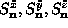to be quantum operators, satisfying the Heisenberg equations of motion: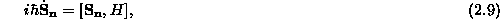with the standard canonical commutators,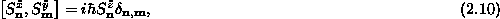and its cyclic permutations. Because we are studying the small amplitude deviations from the static vortex configuration, we need the equations of motion linearized in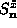and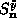, with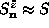. Doing so, we obtain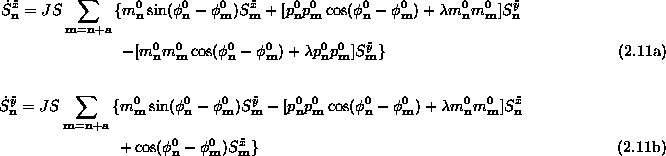Now we look for eigenstates or normal modes, in the sense that we try to find operators which are linear combinations of theandoperators, with a single-frequency time dependence. Or, in quantum language, we look for creation and annihilation operators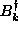and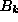in which the Hamiltonian will be a sum of terms in the simple diagonal form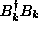, where k is an index that distinguishes the different modes making up a complete set. While the equations are solved for finite systems, the usual momentum is not a good quantum number, due to the lack of translational invariance. But the modes will be distinguished by the effective wavelengths of the standing waves present, and by the locations of nodes and antinodes in the wavefunctions or their squares. In any case, we suppose the modes are ordered in some way, perhaps from largest to smallest frequency, and notated by an index k. Some modes may be energetically degenerate, in which case k must denote more than just the frequency. It is clear that this type of problem will produce pairs of conjugate modes,and, and we suppose these unknown operators are the linear combinations,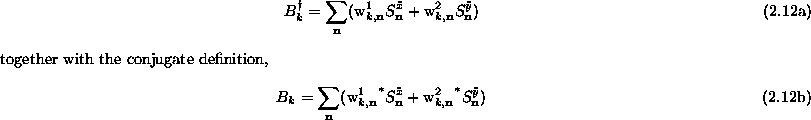where the complex expansion coefficients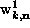and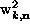are to be determined. (This being a linear problem, there should be no confusion that the superscripts ``1'' and ``2'' are not powers.) With the requirement of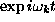time dependence, where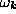is the unknown eigenfrequency to be determined,must satisfy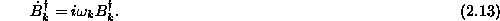Using Eqs. (2.12) and (2.11) in Eq. (2.13) leads to the following matrix equation for the coefficients: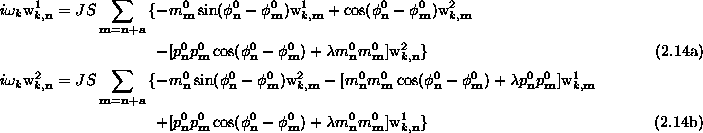For numerical diagonalization, the lattice sites are numbered in some arbitrary order, and then a vector can be formed out of theandvariables as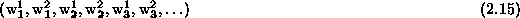This will allow Eqs. (2.14) to be solved numerically for the eigenvaluesand their respective eigenvectors, given in terms of the coefficients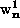and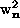. In this notation, the matrix to be diagonalized is real, but not Hermitian.

Once we have the complete set of these normal modes and their eigenfrequencies, the Hamiltonian will be expressed in the diagonal form;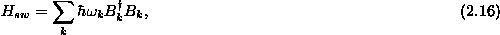whereandhave equal frequencies, but with opposite signs.Next: NORMALIZATIONSPIN EXPECTATIONS Up: PERTURBATION ABOUT A Previous: Figure 2. Local

Gary M Wysin
Mon Sep 11 12:02:10 CDT 1995